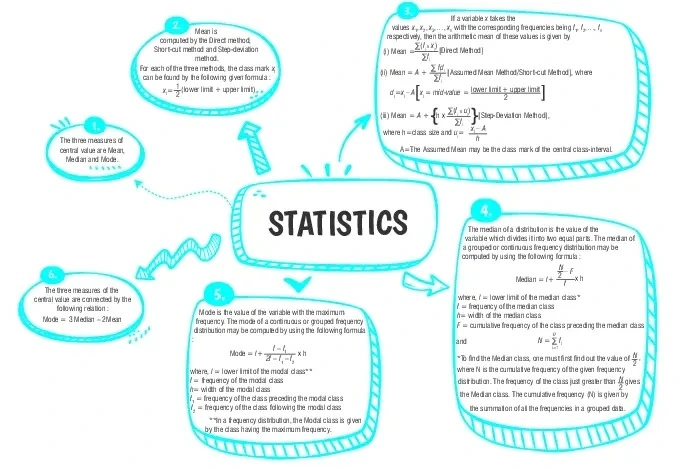Statistics

# Class 10 Math Chapter 13 Statistics Important QuestionsHere are some important questions for Class 10 Mathematics Chapter 13, Statistics, carefully selected to help students prepare effectively for the CBSE Class 10 Mathematics Examination in 2023-24. By practicing these diverse problems, students can improve their understanding of Statistics concepts and enhance their problem-solving abilities. These questions are designed to clarify doubts and contribute to better performance in the Statistics chapter.

## Introduction

In Chapter 13 of Class 10 Mathematics,Statistics, we will focus on understanding and calculating the measures of central tendency—mean, median, and mode—for grouped data. These measures help us analyze and summarize large sets of data by providing insights into the typical values and trends within the data.

### What is the purpose of statistics in mathematics?

Statistics involves collecting, analyzing, and interpreting data to understand trends, patterns, and information in various fields. It helps in making informed decisions and drawing conclusions.### Class 10 Statistics Important Questions and Answers

#### (a) The lower class boundary is the same as the upper class boundary of the previous class.(b) The upper class boundary is the same as the lower class boundary of the next class.(c) Both the lower and upper class boundaries are the same.(d) The lower and upper class boundaries are contained within the class and do not intersect with either the upper boundary of the previous class or the lower boundary of the next class.

Ans. (d)

Explanation:
The lower and upper class boundaries are contained within the class and do not intersect with either the upper boundary of the previous class or the lower boundary of the next class.

#### (a)1.75 and 45(b)17.5 and 4.5(c)1.75 and 4.5(d)17.5 and 45

Ans. (b)17.5 and 4.5

Explanation:
Explanation: We derive the class mark xi by the following formula :

xi=$$\frac{1}{2}$$ (lower limit + upper limit)
Thus, xi=$$\frac{1}{2}$$(10 + 25)
=$$\frac{1}{2}$$(35)=17.5
And xii = $$\frac{1}{2}$$ (lower limit + upper limit)
Thus, xii =$$\frac{1}{2}$$(35 + 55)
=$$\frac{1}{2}$$(90) = 45

#### Q3. The table below shows the distribution of marks obtained by students in an examination. Calculate the value of x if the mean mark is 18.

Marks No. of students
5 6
10 4
15 6
20 12
25 x
30 4

Ans.  x = 8

Explanation:
Given Mean = 18

Marks No. of students
(xi) (fi) fi × xi
5 6 30
10 4 40
15 6 90
20 12 240
25 x 25x
30 4 120
Total 32 + x 520 + 25x

Mean = $$\frac{\Sigma f_ix_i}{\Sigma f_i}$$
18 = 520 + 25 x/32+ x
18(32+x)  = 520 +25x
576-520 = 25x-18x
X = 8

#### Q4. Find the mean of the following frequency distribution:

Class Interval Frequency
0-50 4
50-100 8
100-150 16
150-200 13
200-250 6
250-300 3

Ans. Mean = 143

Explanation:

Class Interval Frequency (fi ) Class Marks
(xi)
(fi xi)
0-50 4 25 100
50-100 8 75 600
100-150 16 125 2000
150-200 13 175 2275
200-250 6 225 1350
250-300 3 275 825
Σfi = 50 (fi xi) =7150

Explanation:
Mean =  Σfixi/Σfi = 7150/50
= 143

#### Q5. In a class of 30 students, the heights (in cm) of the students are recorded as follows: 140, 142, 145, 150, 148, 152, 156, 145, 146, 144, 149, 151, 153, 148, 142, 147, 149, 155, 146, 144, 147, 150, 153, 147, 145, 149, 151, 153, 152, 148. Calculate the mean height of the students.

Ans. 149.2

Explanation:
Sum up all the heights: Sum = 140 + 142 + 145 + 150 + 148 + 152 + 156 + 145 + 146 + 144 + 149 + 151 + 153 + 148 + 142 + 147 + 149 + 155 + 146 + 144 + 147 + 150 + 153 + 147 + 145 + 149 + 151 + 153 + 152 + 148
Sum = 4476
Calculate the mean:
Mean = Sum / Total number of students
Mean = 4476 / 30
Mean = 149.2 cm#### CBSE Class 10 Maths Chapter   wise Important Questions

Chapter No. Chapter Name
Chapter 1 Real Number
Chapter 2 Polynomials
Chapter 3 Pair of Linear Equations in Two Variables
Chapter 5 Arithmetic Progressions
Chapter 6 Triangles
Chapter 7 Coordinate Geometry
Chapter 8 Introduction to Trigonometry
Chapter 9 Some Applications of Trigonometry
Chapter 10 Circles
Chapter 11 Areas Related to Circle
Chapter 12 Surface Areas and Volumes
Chapter 13 Statistics
Chapter 14 Probability

#### Conclusion

To enhance your grasp of the Statistics chapter, consider exploring oswal.io. This website provides a variety of practice questions tailored to facilitate better learning. By engaging with these questions, you can reinforce your understanding of statistical concepts and improve your problem-solving abilities in this important math topic.

#### Q1: Define 'data' and 'raw data.

Ans: Data refers to information or observations collected for analysis. Raw data is the original, unprocessed form of data before any organization or manipulation.

#### Q2: What is statistics?

Ans: Statistics is a branch of mathematics that involves collecting, organizing, analyzing, interpreting, and presenting data. It provides methods for understanding patterns, trends, and relationships in data.

#### Q3: Define median.

Ans: The median is the middle value of a dataset when arranged in ascending or descending order. It divides the data into two equal halves.

#### Q4: Explain the concept of 'grouped data’.

Ans: Grouped data is data that is categorized into intervals or classes. It simplifies data representation when dealing with large datasets.

#### Q5: How is the 'midpoint' of a class interval calculated?

Ans: The midpoint of a class interval is calculated by adding the lower and upper limits of the interval and dividing by 2.

###### Copyright 2022 OSWAL PUBLISHERS Simplifying ExamsPhone:  (+91) 78959 87722
Mail: support@oswalpublishers.in